Practice Exercises on Sets

 1. Which of the following is sets is shown with roster notation? { q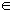| -4 ≤ q < 3 } G = {letters in the English alphabet} X = {red, blue, yellow} None of the above. RESULTS BOX:
 2. Which of the following is true about set D listed below? D = {-3, -2, -1, 0, 1, 2, 3} -7D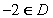D is an infinite set. All of the above. RESULTS BOX:
 3. Which of the following is an infinite set? { x: x  ≥ 2 and x ≤ 6  } A = {letters in the English alphabet} B = {Asia, Africa, North America, South America, Antarctica, Europe, Australia} C = {integers} RESULTS BOX:
 4. Which of the following sets is equal to the set listed below? X = {2, 3, 5, 7, 11, 13, 17, 19} { x|  x ≥ 2 } Y = {prime numbers less than 20} Z = {odd numbers less than 20} All of the above. RESULTS BOX:
 6. If A = {types of triangles} and B = {types of trapezoids}, then which of the following is the universal set?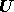= {polygons}= {quadrilaterals}= {hexagons} All of the above. RESULTS BOX:
 7. Which of the following is the correct set-builder notation for the set listed below? {-5, -4, -3, -2, -1, 0, 1, 2, 3, ...} { K| k > -5 } { q| q ≥ -5 } { x| x < 3 } All of the above. RESULTS BOX:
 8. In the Venn diagram below, If= {students who attend The Smart School}, then which of the following is the complement of set A?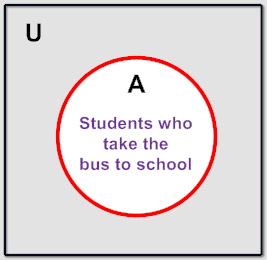A' = {students who do not attend The Smart School} A' = {students who attend the Not So Smart School} A' = {students who do not take the bus to school} None of the above. RESULTS BOX:
 9. Which of the following is represented by the shaded region in the Venn diagram below?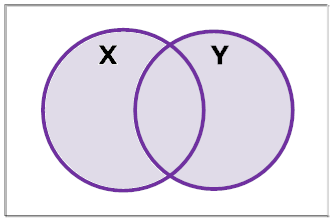X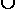Y X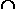Y X' All of the above. RESULTS BOX:
 10. Which of the following is represented by the shaded region in the Venn diagram below?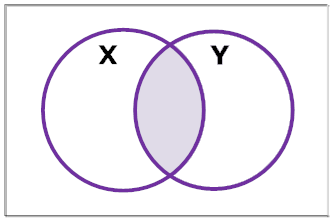XY XY X' All of the above. RESULTS BOX: